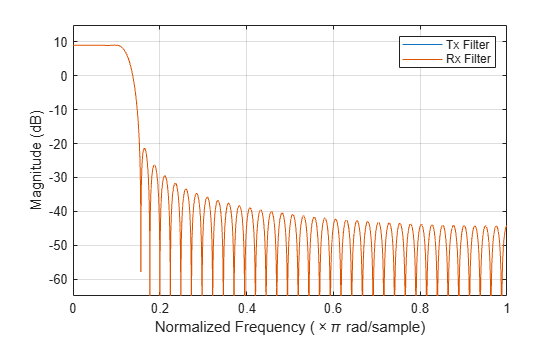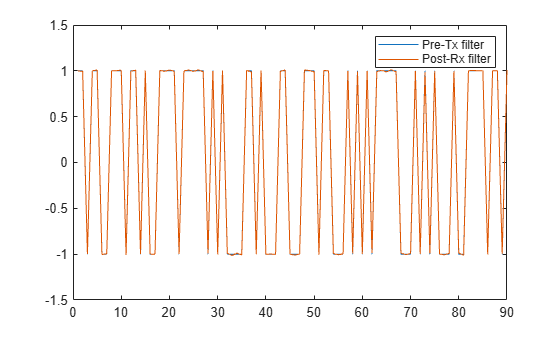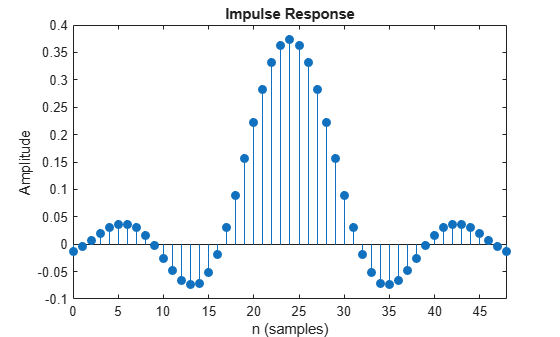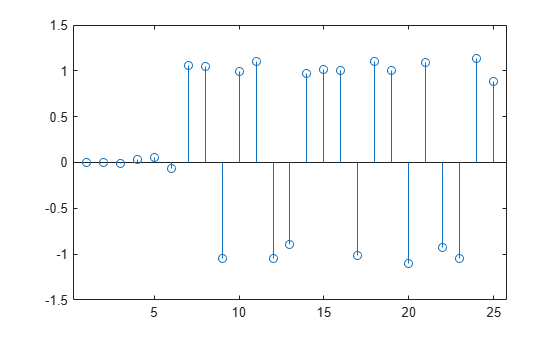Apply pulse shaping by decimating signal using raised-cosine FIR filter

## Description

The `comm.RaisedCosineReceiveFilter` System object™ applies pulse shaping by decimating an input signal using a raised-cosine finite impulse response (FIR) filter. The FIR filter has (`FilterSpanInSymbols` × `InputSamplesPerSymbol` + 1) tap coefficients.

To apply pulse shaping by decimating an input signal using a raised-cosine FIR filter:

1. Create the `comm.RaisedCosineReceiveFilter` object and set its properties.

2. Call the object with arguments, as if it were a function.

## Creation

### Syntax

``rxfilter = comm.RaisedCosineReceiveFilter``
``rxfilter = comm.RaisedCosineReceiveFilter(Name,Value) ``

### Description

````rxfilter = comm.RaisedCosineReceiveFilter` returns a raised-cosine FIR receive filter System object, which decimates the input signal using a raised-cosine FIR filter. The filter uses an efficient polyphase FIR decimation structure and has unit energy.```

example

````rxfilter = comm.RaisedCosineReceiveFilter(Name,Value) ` sets properties using one or more name-value pairs. Enclose each property name in quotes. For example, `comm.RaisedCosineReceiveFilter('RolloffFactor',0.3)` configures a raised-cosine receive filter System object with the roll-off factor set to `0.3`. ```

## Properties

expand all

Unless otherwise indicated, properties are nontunable, which means you cannot change their values after calling the object. Objects lock when you call them, and the `release` function unlocks them.

If a property is tunable, you can change its value at any time.

Filter shape, specified as `'Square root'` or `'Normal'`.

Data Types: `char` | `string`

Roll-off factor, specified as a scalar in the range [0, 1].

Data Types: `double`

Filter span in symbols, specified as a positive integer. The object truncates the infinite impulse response (IIR) of an ideal raised-cosine filter to an impulse response that spans the number of symbols specified by this property.

Data Types: `double`

Input samples per symbol, specified as a positive integer.

Data Types: `double`

Decimation factor, specified as an integer in the range [1, `InputSamplesPerSymbol`]. This value must evenly divide into `InputSamplesPerSymbol`. The sampling rate of the output signal is reduced by the decimation factor such that `length(y)/length(x)` is equal to `DecimationFactor`. For a matrix input signal, the number of input rows must be a multiple of the decimation factor.

Data Types: `double`

Decimation offset, specified as an integer in the range [0, (`DecimationFactor` − 1)]. This property specifies the number of filtered samples the object discards before downsampling.

Data Types: `double`

Linear filter gain, specified as a positive scalar. The object designs a raised-cosine filter that has unit energy and then applies the linear filter gain to obtain final tap coefficient values.

Data Types: `double`

## Usage

### Syntax

``y = rxfilter(x)``

### Description

example

``` `y = rxfilter(x)` applies pulse shaping by decimating an input signal using a raised cosine FIR filter. The output consists of decimated signal values.```

### Input Arguments

expand all

Input signal, specified as a column vector or a Ki-by-N matrix. Ki is the number of input samples per signal channel, and N is the number of signal channels.

For a Ki-by-N matrix input, the object processes columns of the input matrix as N independent channels.

Data Types: `double` | `single`
Complex Number Support: Yes

### Output Arguments

expand all

Output signal, returned as a column vector or a Ko-by-N matrix. Ko is equal to Ki / `DecimationFactor`. Ki is the number of input samples per signal channel, and N is the number of signal channels.

The System object filters each channel over time and generates a Ko-by-N output matrix. The output signal is the same data type as the input signal.

## Object Functions

To use an object function, specify the System object as the first input argument. For example, to release system resources of a System object named `obj`, use this syntax:

`release(obj)`

expand all

 `info` Information about filter System object `coeffs` Coefficients for filters `cost` Computational cost of implementing filter System object `freqz` Frequency response of discrete-time filter `fvtool` Plot frequency response of filter `grpdelay` Group delay response of discrete-time filter `impz` Impulse response of discrete-time filter `order` Order of discrete-time filter System object
 `step` Run System object algorithm `release` Release resources and allow changes to System object property values and input characteristics `reset` Reset internal states of System object

## Examples

collapse all

Filter the output of a square-root-raised-cosine (SRRC) transmit filter by using a matched SRRC receive filter. The input signal has eight samples per symbol.

Create an SRRC transmit filter object, setting the number of output samples per symbol to 8.

` txfilter = comm.RaisedCosineTransmitFilter('OutputSamplesPerSymbol',8);`

Create an SRRC receive filter, setting the number of input samples per symbol to 8 and the decimation factor to 8.

```rxfilter = comm.RaisedCosineReceiveFilter('InputSamplesPerSymbol',8, ... 'DecimationFactor',8);```

Use the `coeffs` function to determine the filter coefficients for both filters.

```txCoef = coeffs(txfilter); rxCoef = coeffs(rxfilter);```

Launch the filter visualization tool and display the magnitude responses of the two filters. The results show that the responses are the same.

``` fvtool(txCoef.Numerator,1,rxCoef.Numerator,1); legend('Tx Filter','Rx Filter')```Generate a random bipolar signal. Interpolate the signal by using the SRRC transmit filter object.

``` preTx = 2*randi([0 1],100,1) - 1; y = txfilter(preTx);```

Decimate the signal by using the SRRC receive filter object.

` postRx = rxfilter(y);`

The filter delay is equal to the filter span. Accounting for the filter delay, adjust the plotted samples to compare the pre-Tx filter signal with the post-Rx filter signal. Because the combined receive and the transmit RRC filters generate a matched filter pair, the two signals overlap one another.

```delay = txfilter.FilterSpanInSymbols; x = (1:(length(preTx)-delay)); plot(x,preTx(1:end-delay),x,postRx(delay+1:end)) legend('Pre-Tx filter','Post-Rx filter')```Decimate a bipolar signal using a square-root-raised-cosine (SRRC) filter whose impulse response is truncated to filter a span of six symbol durations.

Create a SRRC transmit FIR filter, setting the filter span to six symbols. The object truncates the impulse response to six symbols.

`txfilter = comm.RaisedCosineTransmitFilter('FilterSpanInSymbols',6);`

Generate a random bipolar signal . Filter the signal by using the SRRC transmit FIR filter object.

```x = 2*randi([0 1],25,1) - 1; y = txfilter(x);```

Create a matched SRRC receive filter object.

`rxfilter = comm.RaisedCosineReceiveFilter('FilterSpanInSymbols',6);`

Launch the filter visualization tool to show the impulse response of the receive filter.

`fvtool(rxfilter,'Analysis','impulse')`Filter the output signal from the transmit filter by using the matched SRRC receive filter object.

`r = rxfilter(y);`

Plot the interpolated signal. The results show a delay equal to the filter span (six symbols) before data passes through the filter.

`stem(r)`Create a square-root-raised-cosine (SRRC) receive filter object. Use FVTool to plot the filter response. The results show that the linear filter gain is greater than unity. Specifically, the passband gain is more than 0 dB.

```rxfilter = comm.RaisedCosineReceiveFilter; fvtool(rxfilter)```Use the `coeffs` object function to obtain the filter coefficients and adjust the filter gain to unit energy.

`b = coeffs(rxfilter);`

Because a filter with unity passband gain must have filter coefficients that sum to 1, set the linear filter gain to the inverse of the sum of the filter tap coefficients, `b.Numerator`.

`rxfilter.Gain = 1/sum(b.Numerator);`

Verify that the resulting filter coefficients sum to 1.

```bNorm = coeffs(rxfilter); sum(bNorm.Numerator)```
```ans = 1.0000 ```

Plot the filter frequency response. The results now show that the passband gain is 0 dB, which is unity gain.

`fvtool(rxfilter)`## Extended Capabilities

### Functions

Introduced in R2013b